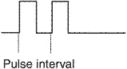# pulse interval

## pulse interval

[′pəls ‚in·tər·vəl]
(physics)

## pulse intervalThe time between consecutive pulses both measured at the same point in the pulse.
References in periodicals archive ?
pneumoniae genomic DNA in phosphate buffer (0.1 mol [L.sup.-1], pH 7.3): modulation amplitude, 25 mV; pulse interval, 0.02 s; scan rate, 50 mV [s.sup.-1].
Surface integrity of machined surface is dependent on various WEDM parameters such as pulse duration, pulse interval, servo voltage, ignition pulse current, wire tension, wire speed and dielectric pressure [6, 7].
And during the pulse interval, the temperature at crater decreases due to thermal conduction.
By using this PPM signal, a microprocessor determines the presence of a metal object by counting the pulse interval. An example showing the effect by active noise reduction is shown in Figure 15.
[E.sub.s] Transmitted power 1 ASNR Average signal noise ratio 8 dB [tau] Temporal correlation 0.1s [M.sub.t] Pulse interval 1 ms [P.sub.fa] False alarm probability 0.05 [P.sub.d] Detection probability 0.95 PAPR Peak to average power ratio 3 dB [f.sub.s] The sampling frequency 10 GHz [f.sub.c] Center frequency of UWB 3 GHz
Mean [+ or -] standard deviation Parameter n (range) Minimum frequency 126 21.67 [+ or -] 1.03 (19.4-24.5) (kHz) Maximum frequency 126 24.67 [+ or -] 1.68 (21.0-30.0) (kHz) Bandwidth (kHz) 126 3.00 [+ or -] 1.17 (-0.34-6.9) Pulse interval (ms) 118 426.6 [+ or -] 163.8 (139-1012) Pulse duration (ms) 104 15.7 [+ or -] 3.3 (9.0-23.0)
The pulse interval was determined as the time from the onset of 1 systolic ejection Doppler waveform across the pulmonary valve to the onset of the next such waveform.
Interaction of blood pressure (BP) and pulse interval (PI) are complex, governed by numerous homeostatic mechanisms, including the autonomic nervous system [1-3].
The heat is duly taken away from the ablation zone of microstructure in the first laser pulse interval so that the heat cannot be further conducted to the workpiece.
Set the parameters as follows: pulse interval is [T.sub.r] = 10 [t.sub.p], the number of pulses is [N.sub.p] = 5, and the range and velocity ambiguity function of coherent pulse train signal are shown in Figure 5.
Resampling with the shortest pulse interval is a simple measure, while it does not take into account the PRI modulation.
It is worthwhile for us to study how to define the basic reproduction ratio for a general system with pulse interval interruption.

Site: Follow: Share:
Open / Close